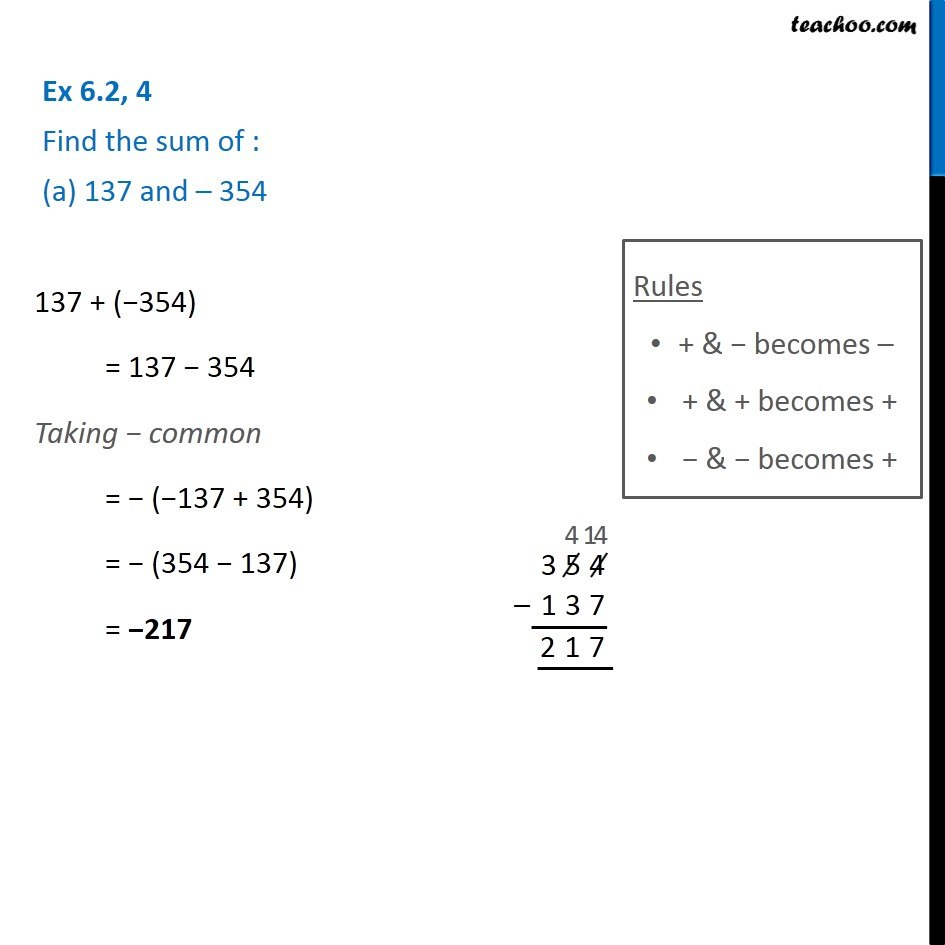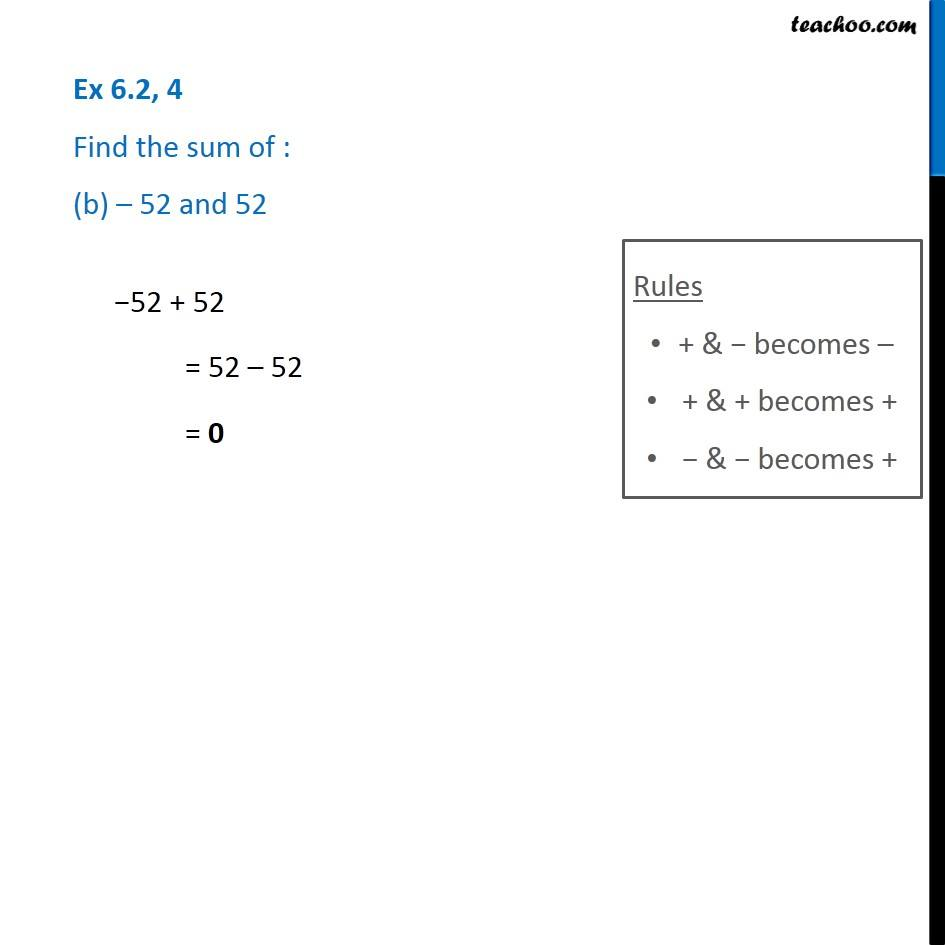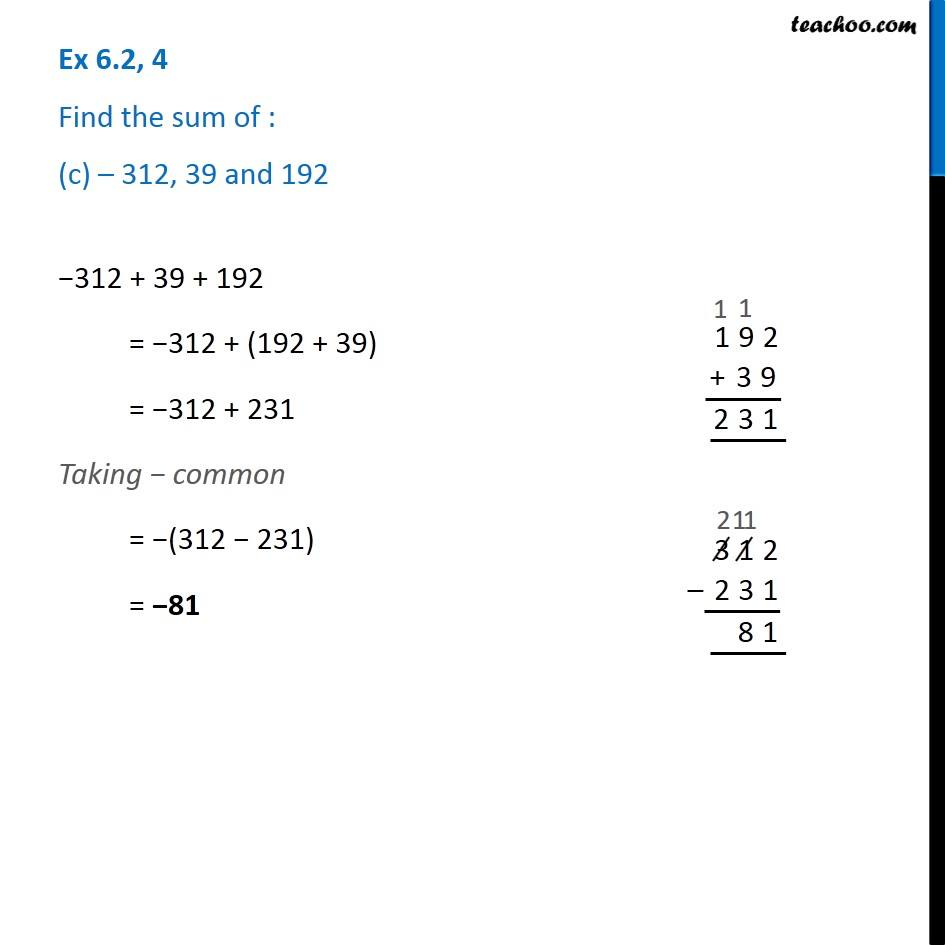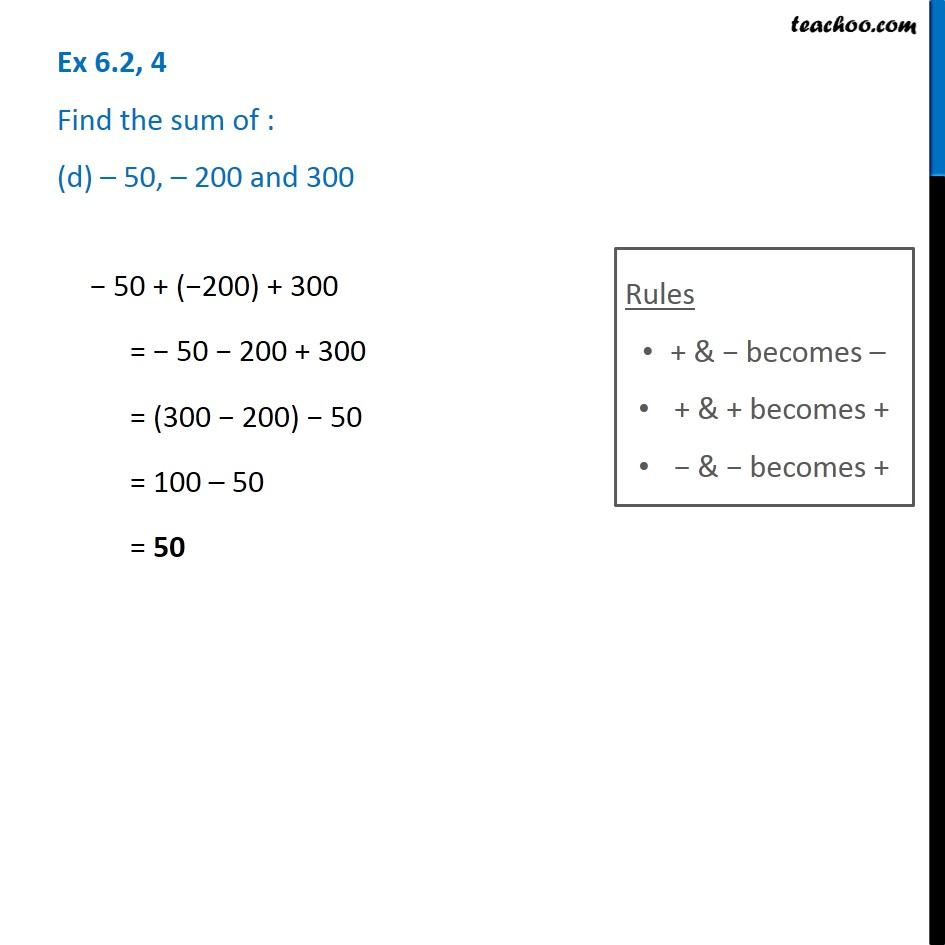Subscribe to our Youtube Channel - https://you.tube/teachoo

1. Chapter 6 Class 6 Integers
2. Serial order wise
3. Ex 6.2

Transcript

Ex 6.2, 4 Find the sum of : (a) 137 and – 354 137 + (−354) = 137 − 354 Taking − common = − (−137 + 354) = − (354 − 137) = −217 Rules + & − becomes – + & + becomes + − & − becomes + Ex 6.2, 4 Find the sum of : (b) – 52 and 52 −52 + 52 = 52 – 52 = 0 Ex 6.2, 4 Find the sum of : (c) – 312, 39 and 192 −312 + 39 + 192 = −312 + (192 + 39) = −312 + 231 Taking − common = −(312 − 231) = −81 Ex 6.2, 4 Find the sum of : (d) – 50, – 200 and 300 − 50 + (−200) + 300 = − 50 − 200 + 300 = (300 − 200) − 50 = 100 – 50 = 50

Ex 6.2

Chapter 6 Class 6 Integers
Serial order wise